# Force Types Definition of Force Drawing the Force

• Slides: 17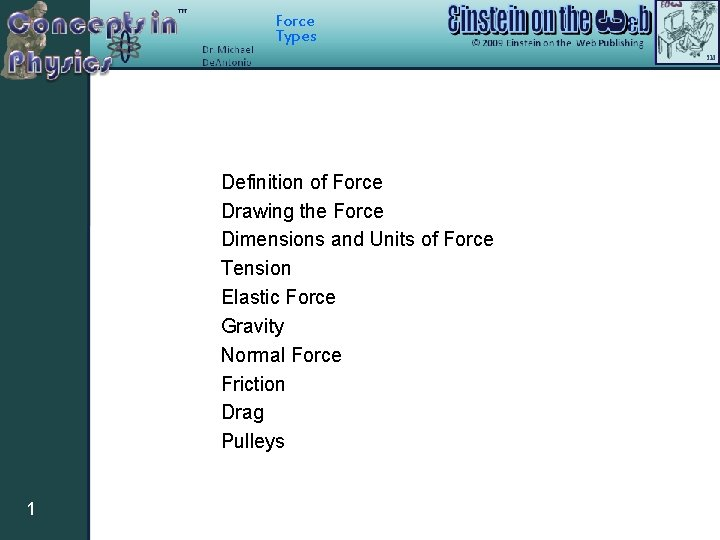Force Types Definition of Force Drawing the Force Dimensions and Units of Force Tension Elastic Force Gravity Normal Force Friction Drag Pulleys 1Force Types Force That which causes a particle to accelerate. It is a vector! Knight’s Definition A force … is a push or a pull on an object. It is a vector. It has both magnitude and direction. It requires an agent. Something does the pushing or pulling. It is either a contact force or a long-range force (gravity). 2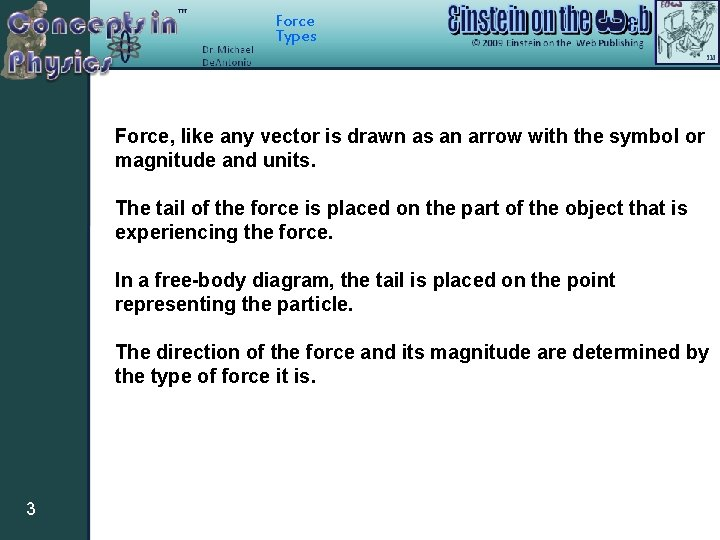Force Types Force, like any vector is drawn as an arrow with the symbol or magnitude and units. The tail of the force is placed on the part of the object that is experiencing the force. In a free-body diagram, the tail is placed on the point representing the particle. The direction of the force and its magnitude are determined by the type of force it is. 3Force Types Force The SI unit of force is the Newton (abbreviated as N). One Newton is the same as one kilogram-meter per second squared. Another unit of force is the pound. 4Force Types Tension The magnitude of the tension is the same everywhere in the rope. 5Force Types Name of Force Tension Symbol 6 When does this force occur? If an object that is experiencing the force is pulling but not stretching another object Where does this force occur? On the object that is experiencing the force at the point where the pulling occurs Associated Energy No associated energyForce Types Spring Force 7Force Types Name of Force Spring Force Symbol 8 When does this force occur? If an object that is experiencing the force is stretching (or compressing) another object Where does this force occur? On the object experiencing the force where it connects to the object that stretches/compresses Associated Energy Elastic Potential Energy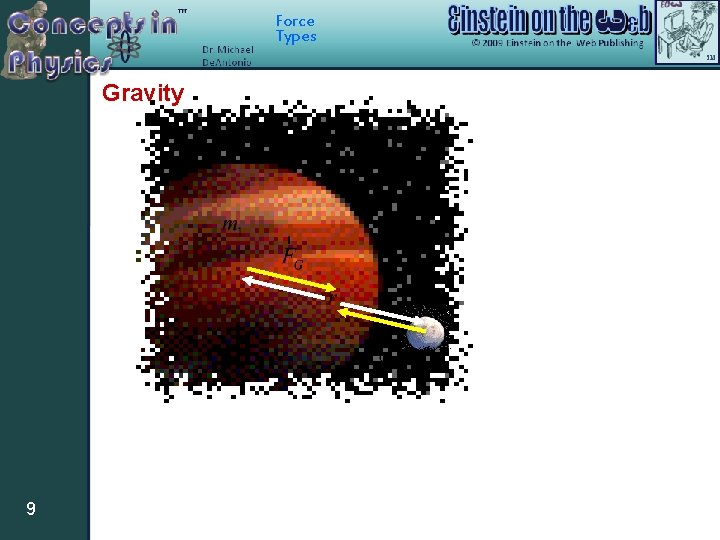Force Types Gravity 9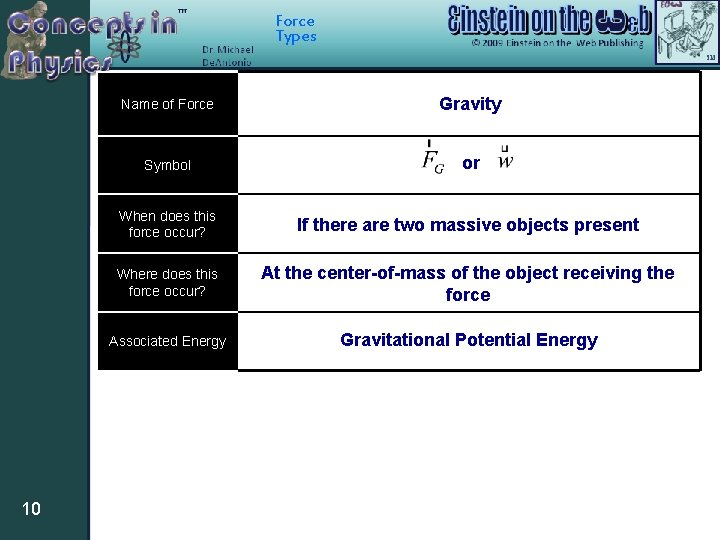Force Types 10 Name of Force Gravity Symbol or When does this force occur? If there are two massive objects present Where does this force occur? At the center-of-mass of the object receiving the force Associated Energy Gravitational Potential Energy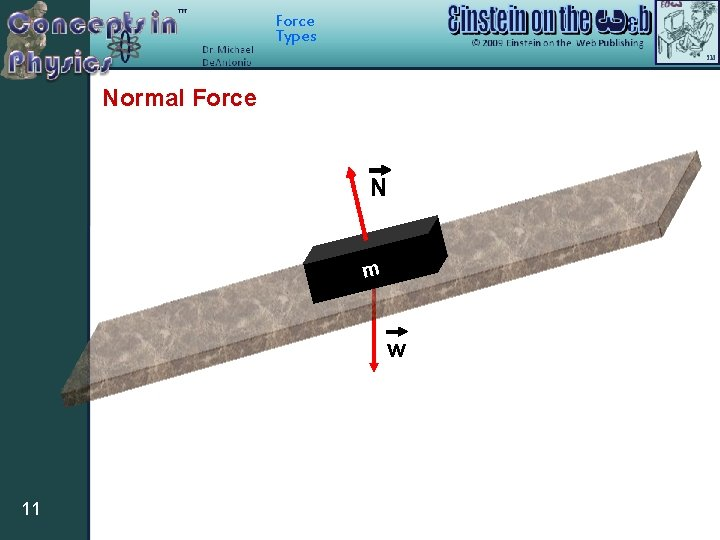Force Types Normal Force N m w 11Force Types 12 Name of Force Normal Force Symbol or When does this force occur? If an object touches the surface of another object Where does this force occur? On the object receiving the force at the point(s) where it touches the surface Associated Energy No associated energyForce Types Friction N n or o i t mo f o n o i t ion c Dire ble mot i poss 13 f m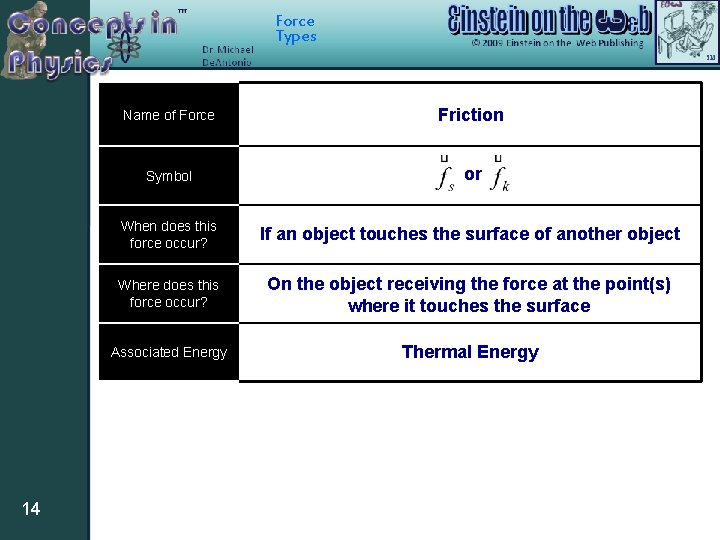Force Types 14 Name of Force Friction Symbol or When does this force occur? If an object touches the surface of another object Where does this force occur? On the object receiving the force at the point(s) where it touches the surface Associated Energy Thermal EnergyForce Types Drag D 15Force Types Name of Force Drag Symbol 16 When does this force occur? If an object is moving through a liquid or gas. Where does this force occur? On the object receiving the force at the point(s) where it touches the liquid or gas Associated Energy Thermal EnergyForce Types Pulleys simply change the DIRECTION of a force. T T 17